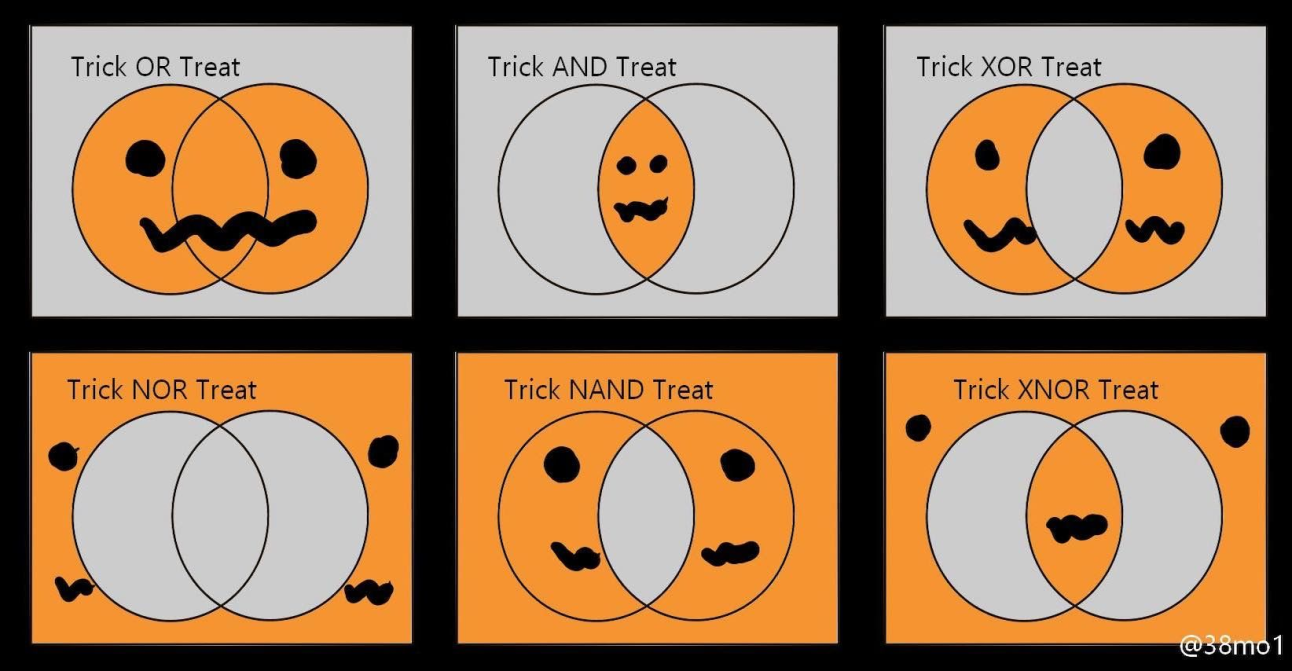• # Eat Cookies, Make a New Math and Dive Into Variables Scope and Functools ModuleHello, checkiomates🐱‍👤!

Did you know, that we allow users to assign hotkeys to "Run Code", "Check Solution" and stop code. You may see current combinations on buttons and change them in editor menu. If you want to discover all CheckiO features, visit our tutorial. It's a longread, but it's worth it!

🏁 MISSIONS:

Your tasks are to eat all 🍪 at the lowest number of steps, redefine addition and multiplication 🧮 in a new way and count clear divisibles! It's not hard yet interesting!

The Cookie Monster by freeman_lex - The beloved Cookie Monster from Sesame Street has stumbled upon a table with sorted piles of cookies, each pile a positive integer. However, the monomaniacal obsessiveness of the Count who set up this crumbly fiesta has recently escalated to a whole new level of severity. The Count insists that these cookies must be eaten in the smallest possible number of moves. Each move chooses one of the remaining pile sizes p, and removes p cookies from every pile that contains at least p cookies (thus eradicating all piles with exactly p cookies), and leaves all smaller piles as they were.

Since the Count also has an unhealthy obsession with order and hierarchies, he expects these moves to be done in decreasing order of values of p. This function should return the list of moves, that allows Cookie Monster to scarf down these cookies. If there are a few optimal sequences of moves, choose the lexicographically largest one. Look at the example for [1, 2, 3, 4, 5, 6] input.

```cookie_monster([1, 2, 3]) == [2, 1]
cookie_monster([1, 2, 3, 4, 5, 6]) == [4, 2, 1]
cookie_monster([2, 3, 5, 8, 13, 21, 34, 55, 89]) == [55, 21, 8, 3, 2]```

"Lunar" Multiply by freeman_lex - Formerly known as “dismal arithmetic”, addition and multiplication of natural numbers are redefined so that adding two digits means taking their maximum, whereas multiplying two digits means taking their minimum. For example, 2 + 7 = 7 + 2 = 7 and 2 × 7 = 7 × 2 = 2. Unlike ordinary addition, there can never be a carry to the next column of digits, no matter how many individual digits are added together in that column. For numbers that consist of several digits, addition and multiplication work exactly as you learned back in grade school, except that the shifted digit columns from lunar multiplication of the individual digits are added in the same lunatic fashion.

```lunar_multiply(2, 3) == 2
lunar_multiply(8, 9) == 8
lunar_multiply(10, 10) == 100```

Count Divisibles in Range by freeman_lex - Given three integers start, end, n, so that start <= end, count how many integers between start and end, inclusive, are evenly divisible by n. Note that either start or end can well be negative or zero, but n is guaranteed to be greater than zero. Here is the scheme for 1, 9, 3 input.

```count_divisibles(7, 28, 4) == 6
count_divisibles(-77, 19, 10) == 9
count_divisibles(1, 999999999999, 5) == 199999999999```

💡ARTICLES:

Today's articles are dedicated to hidden problems in floats comparison, exploring functools module and understanding variables scope.

Python Gotcha: Comparisons - Comparing two numerical variables in Python can have surprising results if you aren’t aware of some common gotchas. This post covers a couple of the common ones.

6 Cool Things You Can Do With The Functools Module - The functools module in the standard library has all sorts of useful bits and pieces. This article talks about six of them: caching, writing fewer dunder methods, freeze functions, generic functions, better decorators, and reduce().

Understanding Python Variables: Namespaces and Variable Scope - This post is a comprehensive guide on namespaces and variable scope. Learn about the four different name spaces and how to access each.

Helloween Logic! 🎃